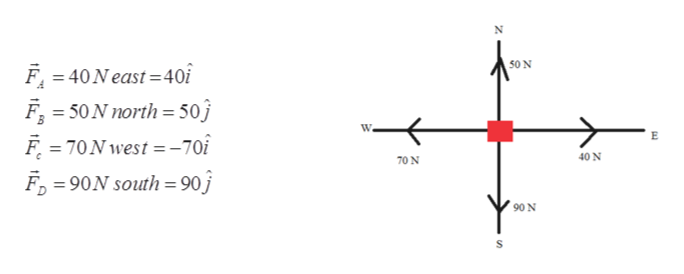# If a TOF mass spectrometer needs to scan an m/z of 600, what is the speed of this ion if it is accelerated through 4000 volts?  How long will this ion (at the speed calculated) take to drift 2.00 m through the spectrometer?  How many mass spectra can be taken each second if a new cycle begins each time this ion reaches the detector?

Question
26 views

If a TOF mass spectrometer needs to scan an m/z of 600, what is the speed of this ion if it is accelerated through 4000 volts?  How long will this ion (at the speed calculated) take to drift 2.00 m through the spectrometer?  How many mass spectra can be taken each second if a new cycle begins each time this ion reaches the detector?

check_circle

Step 1

Given:help_outlineImage Transcriptionclose50 N F, = 40Neast=40i %3D F F = 70N west = -70î = 50 N north = 50j W. 40 N 70 N F, = 90N south = 90 j 90 N fullscreen
Step 2

Net force on the block along x-axis and y – axis can be given as

Step 3

Part (a) Resultant force ca...

### Want to see the full answer?

See Solution

#### Want to see this answer and more?

Solutions are written by subject experts who are available 24/7. Questions are typically answered within 1 hour.*

See Solution
*Response times may vary by subject and question.
Tagged in

### Vectors and Scalars